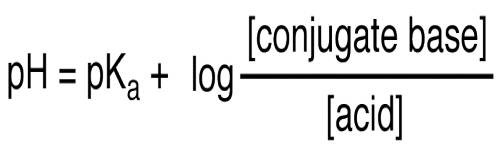Chemistry

# Henderson-Hasselbalch EquationThe Henderson-Hasselbalch equation

The pH of an acidic or basic buffer can be calculated by using the Henderson-Hasselbalch equation. The Henderson-Hasselbalch equation can also be used to prepare a buffer solution of specific pH. In order to derive this equation let us consider the pH of a buffer solution containing a weak acid HA and a soluble salt NaA of the weak acid. In solution, the dissociation of weak add HA can be written as-

HA (aq) ↔ H+ (aq)+ A (aq)

The acid dissociation constant Ka is given by

Ka = [H+][A]/ [HA]

or, [H+] = Ka[HA] / [A] … … …. (1)

Taking logarithm of both sides of equation (1) and multiplying by -1 we obtain,

– log [H+] = – log Ka – log {[HA] / [A]}}

or, – log [H+] = – log Ka + log {[A] / [HA]}

or, pH = pKa + log {[A] / [HA]} … … (2)

Equation (2) is known as the Henderson-Hasselbalch equation.

pH = pKa + log ([A-]/[HA])

[A-] = molar concentration of a conjugate base

[HA] = molar concentration of an undissociated weak acid (M)

The equation can be rewritten to solve for pOH:

pOH = pKb + log ([HB+]/[ B ])

[HB+] = molar concentration of the conjugate base (M)

[ B ] = molar concentration of a weak base (M)In this equation [HA] can be considered to be equal to the initial concentration of HA as this is a weak acid. The added salt farther diminishes its dissociation because of the presence of the common ion A (common ion effect), mains it un-dissociated for all practical purposes. The Henderson-Hasselbalch equation is valid when it contains equilibrium concentrations of an acid and a conjugate base. In the case of solutions containing not-so-weak acids (or not-so-weak bases) equilibrium concentrations can be far from those predicted by the neutralization stoichiometry.